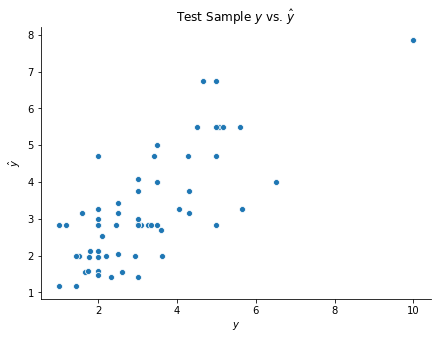# Implementation¶

This section demonstrates how to fit regression and decision trees with scikit-learn. We will again use the tips dataset for the regression tree and the penguins dataset for the classification tree.

## Import packages
import numpy as np
import matplotlib.pyplot as plt
import seaborn as sns
import pandas as pd


## 1. Regression Tree¶

Let’s start by loading in the data. We’ll keep things as pandas dataframes rather than numpy arrays now.

## Load tips data
X = tips.drop(columns = 'tip')
y = tips['tip']

## Train-test split
np.random.seed(1)
test_frac = 0.25
test_size = int(len(y)*test_frac)
test_idxs = np.random.choice(np.arange(len(y)), test_size, replace = False)
X_train = X.drop(test_idxs)
y_train = y.drop(test_idxs)
X_test = X.loc[test_idxs]
y_test = y.loc[test_idxs]


We can then fit the regression tree with the DecisionTreeRegressor class. Unfortunately, scikit-learn does not currently support categorical predictors. Instead, we have to first convert these predictors to dummy variables. Note that this implies that splits of categorical variables can only separate one value from the rest. For instance, a variable with discrete values $$a, b, c,$$ and $$d$$ could not be split as $$\{a, b\}$$ versus $$\{c, d\}$$.

from sklearn.tree import DecisionTreeRegressor

## Get dummies
X_train = pd.get_dummies(X_train, drop_first = True)
X_test = pd.get_dummies(X_test, drop_first = True)

## Build model
dtr = DecisionTreeRegressor(max_depth = 7, min_samples_split = 5)
dtr.fit(X_train, y_train)
y_test_hat = dtr.predict(X_test)

## Visualize predictions
fig, ax = plt.subplots(figsize = (7, 5))
sns.scatterplot(y_test, y_test_hat)
ax.set(xlabel = r'$y$', ylabel = r'$\hat{y}$', title = r'Test Sample $y$ vs. $\hat{y}$')
sns.despine()## 2. Classification Tree¶

The classification tree implementation in scikit-learn is nearly identical. The corresponding code is provided below.

## Load penguins data
penguins = penguins.dropna().reset_index(drop = True)
X = penguins.drop(columns = 'species')
y = penguins['species']

## Train-test split
np.random.seed(1)
test_frac = 0.25
test_size = int(len(y)*test_frac)
test_idxs = np.random.choice(np.arange(len(y)), test_size, replace = False)
X_train = X.drop(test_idxs)
y_train = y.drop(test_idxs)
X_test = X.loc[test_idxs]
y_test = y.loc[test_idxs]

from sklearn.tree import DecisionTreeClassifier

## Get dummies
X_train = pd.get_dummies(X_train, drop_first = True)
X_test = pd.get_dummies(X_test, drop_first = True)

## Build model
dtc = DecisionTreeClassifier(max_depth = 10, min_samples_split = 10)
dtc.fit(X_train, y_train)
y_test_hat = dtc.predict(X_test)

## Observe Accuracy
np.mean(y_test_hat == y_test)

0.9036144578313253